Function Repository Resource:

# ConsistentAugmentedMatrixQ

Determine if a matrix represents a consistent system of linear equations

Contributed by: Peter Cullen Burbery
 ResourceFunction["ConsistentAugmentedMatrixQ"][matrix] returns True if matrix represents a consistent system of linear equations and returns False otherwise.

## Details

The function uses MatrixRank and the coefficient matrix to verify the rank of the coefficient matrix equals the rank of the augmented matrix.
The input should be an augmented matrix representing a system of linear equations.
The function is based on the Rouchè-Capelli theorem.

## Examples

### Basic Examples

Determine if an augmented matrix represents a consistent linear system of equations:

 In:=Out=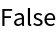The reduced row echelon form contains a contradiction that 0x1+0x2+0x3=1 so the matrix is not consistent:

 In:=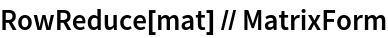Out=The solution set is empty. No solutions exist:

 In:=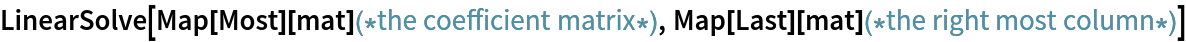Out=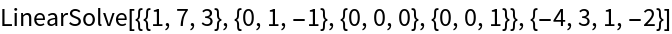In:=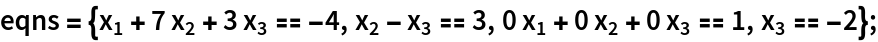In:=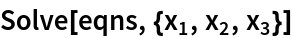Out=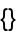The following augmented matrix represents a consistent linear system:

 In:=Out=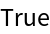Peter Burbery

## Version History

• 1.0.0 – 19 December 2022